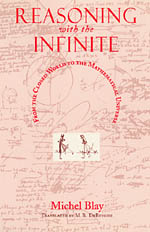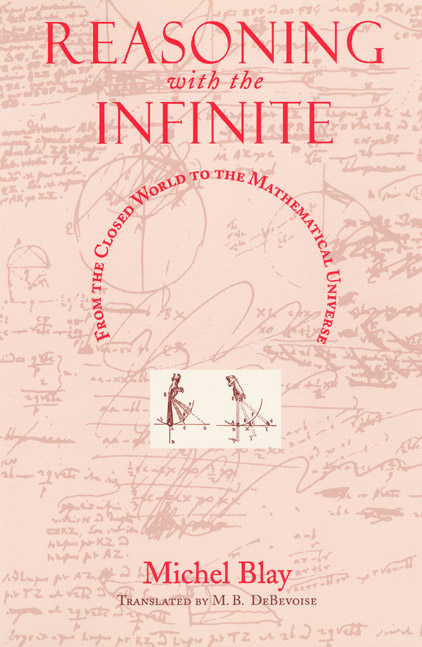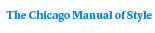# Reasoning with the Infinite

## From the Closed World to the Mathematical Universe

Michel BlayTranslated by M. B. DeBevoise
226 pages | 26 line drawings | 6 x 9 | © 1998
Paper \$28.00 ISBN: 9780226058351 Published November 1999
Until the Scientific Revolution, the nature and motions of heavenly objects were mysterious and unpredictable. The Scientific Revolution was revolutionary in part because it saw the advent of many mathematical tools—chief among them the calculus—that natural philosophers could use to explain and predict these cosmic motions. Michel Blay traces the origins of this mathematization of the world, from Galileo to Newton and Laplace, and considers the profound philosophical consequences of submitting the infinite to rational analysis.

"One of Michael Blay’s many fine achievements in Reasoning with the Infinite is to make us realize how velocity, and later instantaneous velocity, came to play a vital part in the development of a rigorous mathematical science of motion."—Margaret Wertheim, New Scientist

Table of Contents• Contents
Contents
Translator’s Note
Introduction
1: Infinity Eliminated; or, Huygens’s Theory of the Motion of Heavy Bodies
1: Establishing the General Fact of Gravity
2: Mathematical Speculations about Curvilinear Falls
3: The Deductive Scheme of the Science of Motion of Heavy Bodies
2: First and Last Ratios in the Newtonian Theory of Central Forces
1: The Construction of Circular Motion
2: Mechanist Interlude: Centrifugal Force and Weight
3: The Deductive Scheme of Newton’s Principia
3: The Science of Motion in the Workshops of Infinity
1: Satisfying Reason
2: Ratios of the Beginnings, Ends, and Continuous Evolution of Motions
4: Motion Algorithmized
1: Introduction and Import of the Leibnizian Calculus
2: The New Algorithmic Science of Motion
Epilogue: Fontenelle and the Reasons of Infinity
1: The Mathematics of Infinity
2: Mathematical Physics and the Rationalization of Infinites
Notes
Bibliography
Index